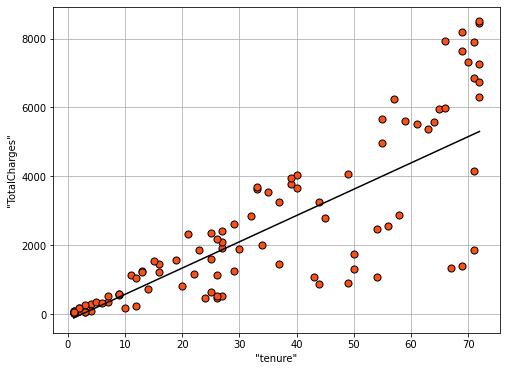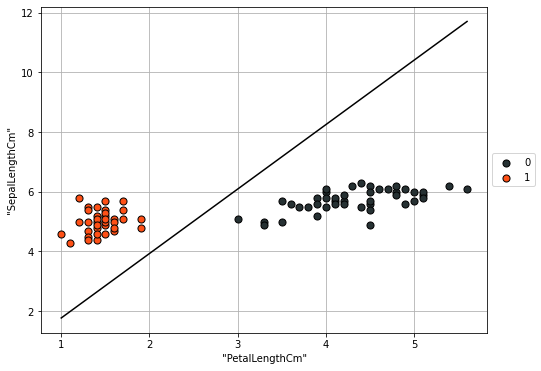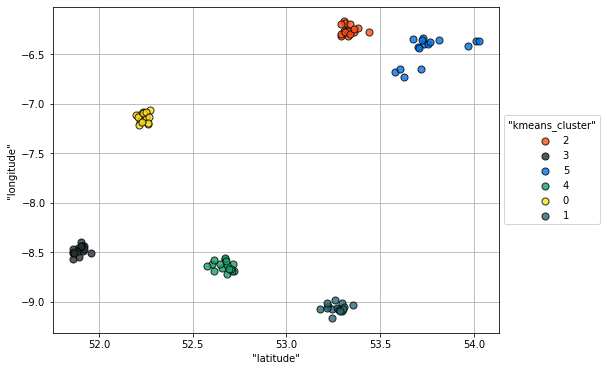VerticaPy

Python API for Vertica Data Science at Scale

Introduction to Machine Learning¶

One of the last stages of the data science life cycle is the Data Modeling. Machine learning algorithms are a set of statistical techniques that build mathematical models from training data. These algorithms come in two types:

• Supervised : these algorithms are used when we want to predict a response column.
• Unsupervised : these algorithms are used when we want to detect anomalies or when we want to segment the data. No response column is needed.

Supervised Learning¶

Supervised Learning techniques map an input to an output based on some example dataset. This type of learning consists of two main types:

• Regression : The Response is numerical (Linear Regression, SVM Regression, RF Regression...)
• Classification : The Response is categorical (Gradient Boosting, Naive Bayes, Logistic Regression...)

For example, predicting the total charges of a Telco customer using their tenure would be a type of regression. The following code is drawing a linear regression using the 'TotalCharges' as a function of the 'tenure' in the Telco Churn Dataset.

In :
from verticapy.learn.linear_model import LinearRegression
model = LinearRegression("LR_churn")
model.drop()
model.fit("churn", ["tenure"], "TotalCharges")
model.plot()
Out:
<AxesSubplot:xlabel='"tenure"', ylabel='"TotalCharges"'>In contrast, when we have to predict a categorical column, we're dealing with classification.

In the following example, we use a Linear Support Vector Classification (SVC) to predict the species of a flower based on its petal and sepal lengths.

In :
from verticapy.learn.svm import LinearSVC
model = LinearSVC("svc_setosa_iris")
model.drop()
model.fit("iris_clean", ["PetalLengthCm", "SepalLengthCm"], "Species_Iris-setosa")
model.plot()
Out:
<AxesSubplot:xlabel='"PetalLengthCm"', ylabel='"SepalLengthCm"'>When we have more than two categories, we use the expression 'Multiclass Classification' instead of 'Classification'.

Unsupervised Learning¶

These algorithms are to used to segment the data (k-means, DBSCAN, etc.) or to detect anomalies (Local Outlier Factor, Z-Score Techniques...). In particular, they're useful for finding patterns in data without labels. For example, let's use a k-means algorithm to create different clusters on the Smart Meters dataset. Each cluster will represent a region.

In :
from verticapy.learn.cluster import KMeans
model = KMeans("KMeans_sm", n_cluster = 6)
model.drop()
model.fit("sm_meters", ["latitude", "longitude"])
model.plot()
Out:
<AxesSubplot:xlabel='"latitude"', ylabel='"longitude"'>In this section, we went over a few of the many ML algorithms available in VerticaPy. In the next lesson, we'll cover creating a regression model.